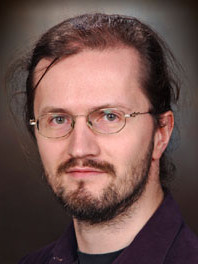# Valentine Kabanets (Theory Seminar S13)"Mining Circuit Lower Bound Proofs for Meta-Algorithms"
Valentine Kabanets
Simon Fraser University
Monday, August 12st, 2013, 2:00 pm
EBU3B, Room 4217
Abstract:

We show that circuit lower bound proofs based on the method of random restrictions yield non-trivial \emph{compression algorithms} for easy'' Boolean functions from the corresponding circuit classes. The compression problem is defined as follows: given the truth table of an $n$-variate Boolean function $f$ computable by some \emph{unknown small circuit} from a \emph{known class} of circuits, find in deterministic time $\poly(2^n)$ a circuit $C$ (no restriction on the type of $C$) computing $f$ so that the size of $C$ is less than the trivial circuit size $2^n/n$. We get non-trivial compression for functions computable by $\AC^0$ circuits, (de Morgan) formulas, and (read-once) branching programs of the size for which the lower bounds for the corresponding circuit class are known.

These compression algorithms rely on the structural characterizations of easy'' functions, which are useful both for proving circuit lower bounds and for designing meta-algorithms'' (such as Circuit-SAT). For (de Morgan) formulas, such structural characterization is provided by the shrinkage under random restrictions'' results~\cite{Sub61,Has98-shrinkage}, strengthened to the high-probability'' version by~\cite{San10,IMZ12,KR12}. We give a new, simple proof of the high-probability'' version of the shrinkage result for (de Morgan) formulas, with improved parameters.  We use this shrinkage result to get  both compression and \#SAT algorithms for (de Morgan) formulas of size about $n^2$. We also use this shrinkage result to get an alternative proof of the recent result by Komargodski and Raz~\cite{KR12} of the average-case lower bound against small (de Morgan) formulas.

Finally, we show that the existence of any non-trivial compression algorithm for a circuit class $\mathcal{C}\subseteq\P/\poly$ would imply the circuit lower bound $\NEXP\not\subseteq \mathcal{C}$. This complements Williams's result~\cite{Wil10} that any non-trivial Circuit-SAT algorithm for a circuit class $\mathcal{C}$ would imply a superpolynomial lower bound against $\mathcal{C}$ for a language in $\NEXP$.

(based on joint work with Ruiwen Chen, Antonina Kolokolova, Ronen Shaltiel, and David Zuckerman)